# Products# Current sensing

## Principle of current sensing

When current is applied to a resistor, the voltage drops at both end of the resistor according to Ohm's law. Dividing the voltage value by the resistance value gives the current value applied to the resistor. If the resistance value is increased to obtain a large voltage drop, the resistor will overheat. To avoid this, a resistor with smallest resistance possible should be used, but when the voltage drop is small, the voltage detection range must be amplified by an amplifier circuit.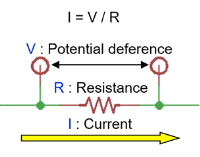## Purposes of current sense resistors

Followings are three applications of current sensing in electrical circuits.

### Overcurrent sensing

A circuit operation needs to be stopped for safety when an overcurrent flows due to a circuit malfunction or overload. If the current exceeds the set value, the resistor judges it as abnormal and interrupt the output.

### Current control

A resistor detects the peak of sawtooth current flowing through the reactor of DC-DC converters and uses it for control.
Also, since the phase and time of the current should be controlled precisely to rotate a three-phase motor, the resistor detects the current flowing in each phase and gives the feedback to the control circuit. Used mainly for electric power steering (EPS) and air conditioners.

### Current management (Battery management)

Devices that operate on secondary batteries sensing current and voltage applied to the battery and optimize circuit operation to enable the ascertaining of battery life and the long-time operation of devices.

Purpose How it works Application

Overcurrent detection

Stops the circuit operation for safety
when an overcurrent flows due to circuit malfunctions.

• Overcurrent protection of power supply circuits
• Overdischarge/overcharge protection of secondary batteries
• Overcurrent protection of motors

Current control

Sense current and gives feedback to a control circuit in order to control the value, duration and phase of the current that flows through the circuit.

• DC-DC converters
•  Inverter power supplies
• Current control of AC motors

Current management

Sense charging/discharging current of a secondary battery in real time to ascertain the battery life and optimally operate the power supply circuit.

• Current management of devices that are powered by secondary batteries such as laptops and mobile devices
• Current management of secondary batteries used in EVs/HEVs

## Categories of current sense resistors

Generally, the resistance of current sense resistors should decrease the voltage from a few 10 mV to a few 100 mV when current flows. Therefore, a low resistance value of several ohms or less is used for current sensing. A type of resistor to be used depends on the current value to be detected.
To detect large current such as a few 10 A, since very low resistance value of a few mΩ is required, a metal plate type or metal foil type that is good at low resistance value is mainly used.

Also, when detecting small current, a thick film type is suitable because relatively large resistance value of several 100 mΩ to several Ω is used.

Click here for our product lineup of current sense low ohm resistors.

Leaded metal film type resistors,  MFMOSX series, are also used for overcurrent detection applications.

## Precautions for sense large current

When sensing large current, current sense resistors use very small resistance value of a few mΩ to reduce the loss caused by the resistor. In order to detect the current accurately, the following precautions must be taken.

• Layout of the voltage terminal pattern
• Inductance of the low ohm resistor (when the temporal di/dt change of current is large)

### Voltage terminal pattern design

To measure the voltage drop across a resistor, the layout of voltage sensing patterns should be designed from both side of the resistor.
Voltage sensing pattern is recommended to be designed from the center of electrode pad of the resistor as shown in fig. (2). This is to avoid the influence from the voltage drop caused by small resistance of the Cu foil pattern on the circuit board.
If the voltage sensing pattern is designed from the side of the electrode pads as shown in fig. (1), the detected voltage drop is under the influence of the resistance of low ohm resistor and Cu foil pattern, which makes current sensing imprecise.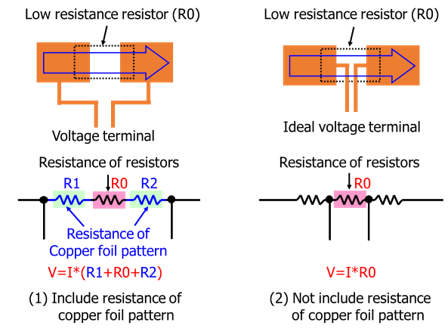### Effect of inductance in low resistance resistors

Resistors are more likely to be affected by parasitic inductance when the resistance is low, and the impedance increases when the frequency gets higher. DC current do not get any influence from this, but AC current such as  sawtooth wave that contains high frequency components get imprecise detection results.
When AC current flows as shown in the figure below, the voltage drop of the resistor is the total sum of the one by resistance and the one by inductance. Thus, the low resistance resistor with minimum inductance should be used for large current sensing.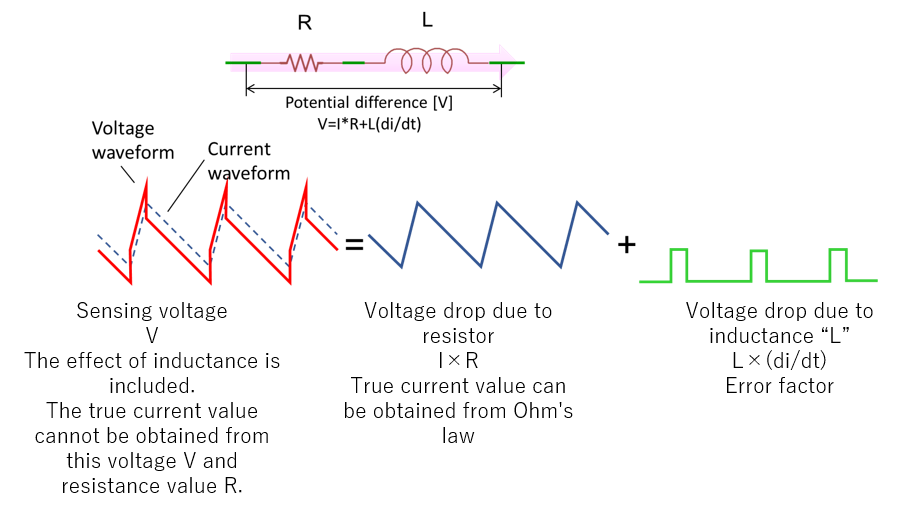## 4-terminal type current sense resistors

Current sense low resistance resistors are available in 4-terminal type with voltage terminals for sensing voltage and current terminals for flowing current. Shown below is an example of 4-terminal type current sense low resistance resistors.
4-terminal types, which have voltage terminals, give an advantage of better accuracy on the current sensing with less sensing errors caused by the voltage terminal pattern design. However, 2-terminal types can also accurately sense current if the voltage terminal patterns are properly designed.
The disadvantage of 4-terminal types is that the overall resistance value is larger than that of 2-terminal types because of the structure, which creates resistance between the voltage terminal and the current terminal in addition to the resistance for sensing current. Therefore, compared with 2-terminal types, the power consumption and the heat generated by the resistor when the same current is applied are larger. When a resistance value is at several mΩ to detect a large current, more power is wasted as the influence of the resistance between the voltage terminal and the current terminal is larger. In addition, since the parasitic inductance is large due to the structure, it is not suitable for sensing current with a large current change (di/dt.)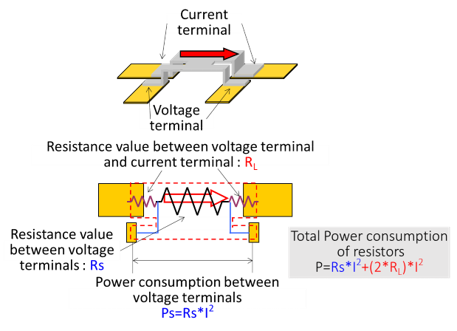## Overcurrent detection (e.g.)

Current sense low resistance resistors are sometimes used for transistor source[space](or emitter) to detect overcurrent of switching transistor. This resistor is required to be free of smoking or starting fire even if the transistor fails, is short circuited and have large current flown in. Also, low inductance is required. For such applications, we recommend metal type low resistance resistors BPR, SL, TSL, LR.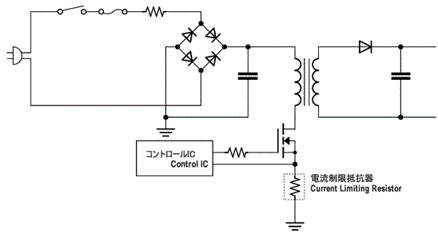Click here for the lineup of current sense resistors and power shunts